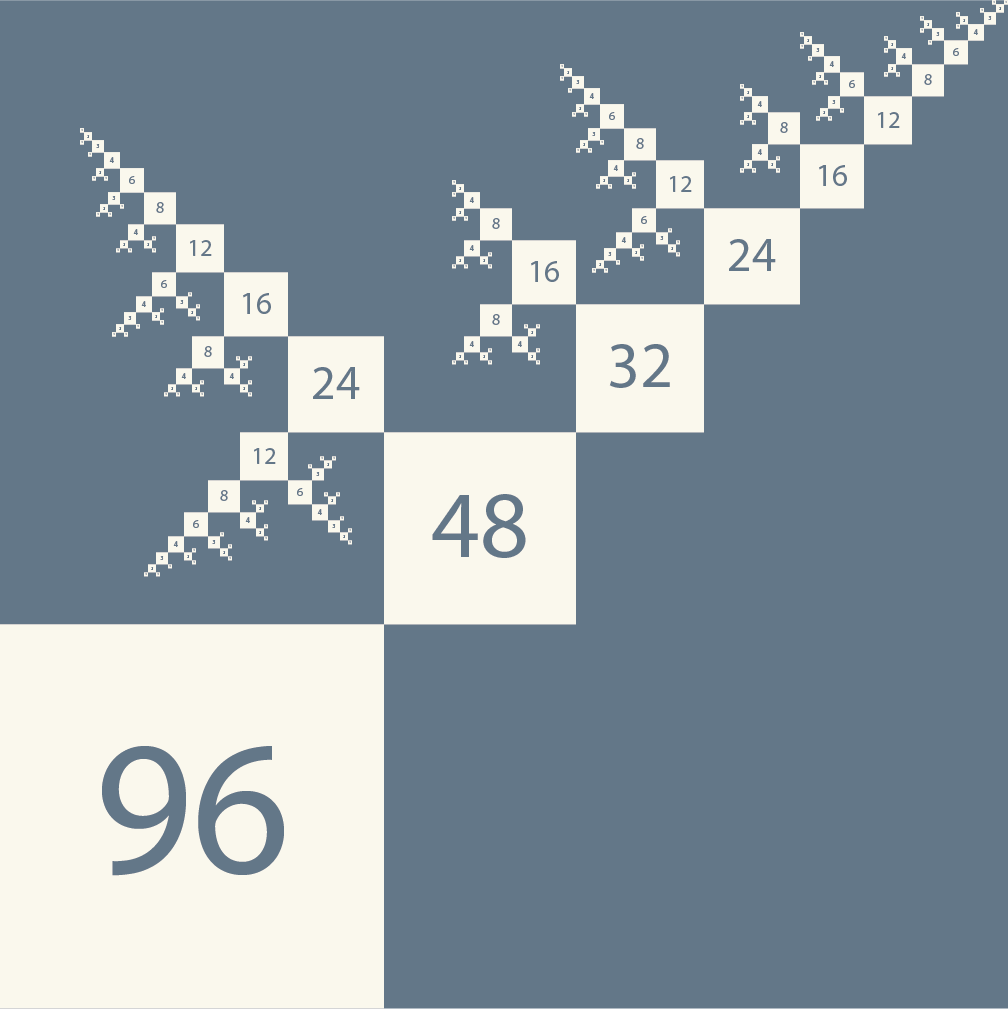# Recursivedivisor properties

Number theory

## Properties of the recursive divisor function and the number of ordered factorisations

Arxiv (2023)

T. Fink

We recently introduced the recursive divisor function $\kappa_x(n)$. Here we calculate its Dirichlet series, which is ${\zeta(s-x)}/(2 - \zeta(s))$. We show that $\kappa_x(n)$ is related to the ordinary divisor function by $\kappa_x * \sigma_y = \kappa_y * \sigma_x$, where * denotes the Dirichlet convolution. Using this, we derive several relations between $\kappa_x(n)$ and standard arithmetic functions, and clarify the relation between $\kappa_0(n)$ and the number of ordered factorisations.

Arxiv (2023)

T. Fink